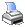﻿ Excel Spreadsheet Features > How to Create Complicated Formulae
 Navigation:  Excel Spreadsheet Features > How to Create Complicated FormulaeOnce there is a mistake in your formula, you will see .Null. values in your column cells. If your formula are not a simple one, you may not be able to easily find out the cause of the problem. In general, when creating a complicated formula, we will follow the following rules.

 • Rather than building the complete formula in one time, you should write a simple part of it first.
 • If the simple part returns correct values, you will then add more to the formula and do the test again.
 • This add and test process should be repeat and repeat until a complete formula is formed.